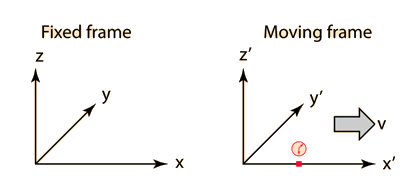iSoul In the beginning is reality.

# Length contraction and time dilation

These derivations follow that in ‘Hyperphysics’ here.

Length Contraction

The length of any object in a moving frame will appear foreshortened in the direction of motion, or contracted. The length is maximum in the frame in which the object is at rest.If the length L0 = x2´ − x1´ is measured in the moving reference frame, then L = x2x1 in the rest frame can be calculated using the Lorentz transformation.

But since the two measurements made in the fixed frame are made simultaneously in that frame, t2 = t1, the expression reduces to:

So length in a moving frame is contracted compared with the same length in a fixed (rest) frame.

Time Dilation

A clock in a moving frame will be seen to be running slow, or “dilated” according to the Lorentz transformation. The time will always be shortest as measured in its rest frame. The time measured in the frame with the clock at rest is called the “proper time”.If the time interval T0 = t2´ − t1´ is measured in the moving reference frame, then T = t2t1 can be calculated using the Lorentz transformation.

But since the two time measurements made in the moving frame are made at the same location (co-located) in that frame, x2 = x1, the expression reduces to:

So a time interval in a moving frame is dilated compared with the same time interval in a fixed (rest) frame.